# Processing IDE with LED On and OFF.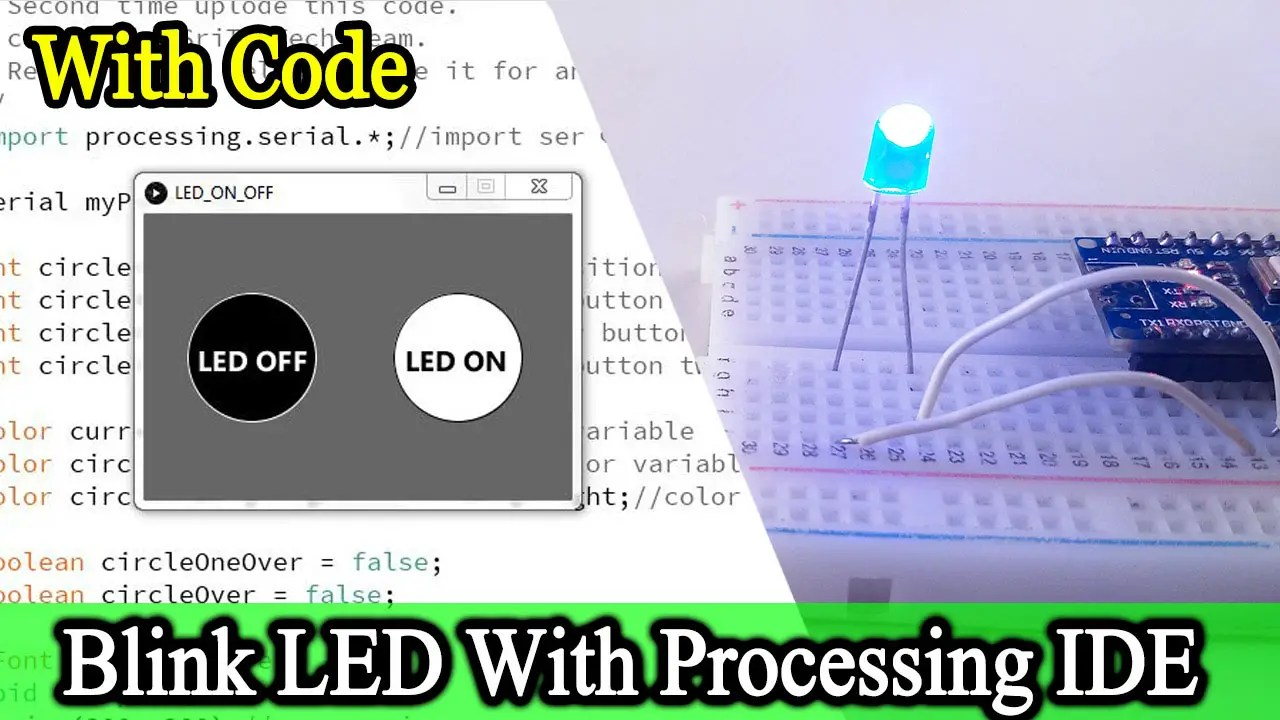Hello guys, welcome back. Today we will learn to On and off an LED bulb using processing IDE. For that, we mainly need the Arduino IDE and Processing IDE. We all know about Arduino IDE. But, Processing IDE is a new thing to our blog. Are you a new member of my blog? Please go to the previous posts and read them. OK, let’s look at today’s post. The first time, we will learn what is the Processing IDE.

### What is the Processing IDE?

Processing IDE is simulation software. This software began development in 2001. Also, this software mainly focused on visualization technology. You can use this software for game development, robot development, and research, etc. Also, we can do 2D and 3D simulations using this software. Examples are drone simulation and flight simulation. However, we need the Java programming language to work with this processing IDE. But, Java’s basic knowledge is enough to work with this software. OK, now let’s go to install the processing IDE on your computer. Let’s do this step by step.

### Step 2

Then, extract this zip file to a location of your choice.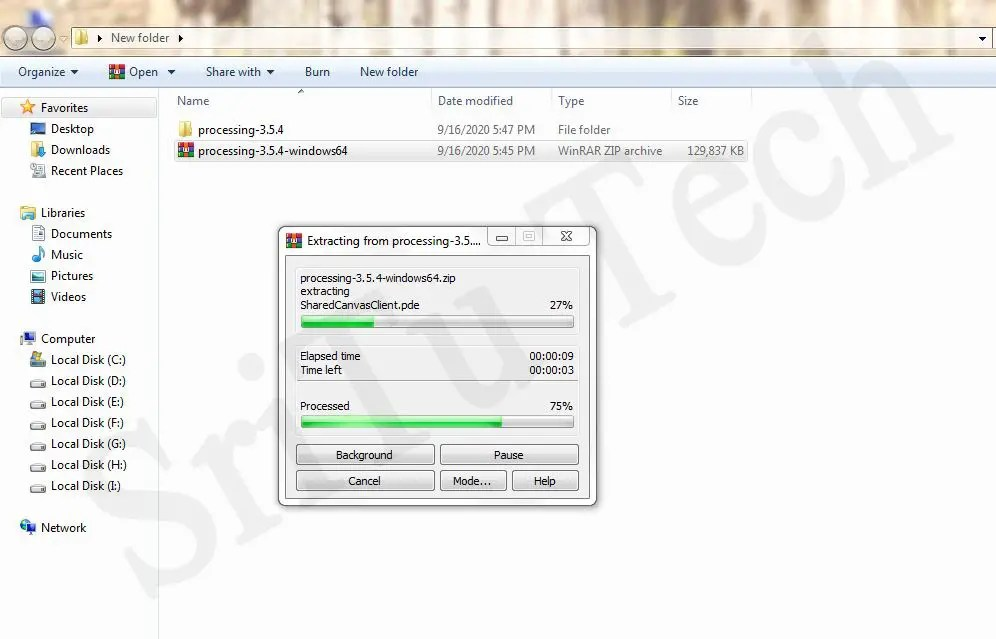### Step 3

Finally, run the processing IDE.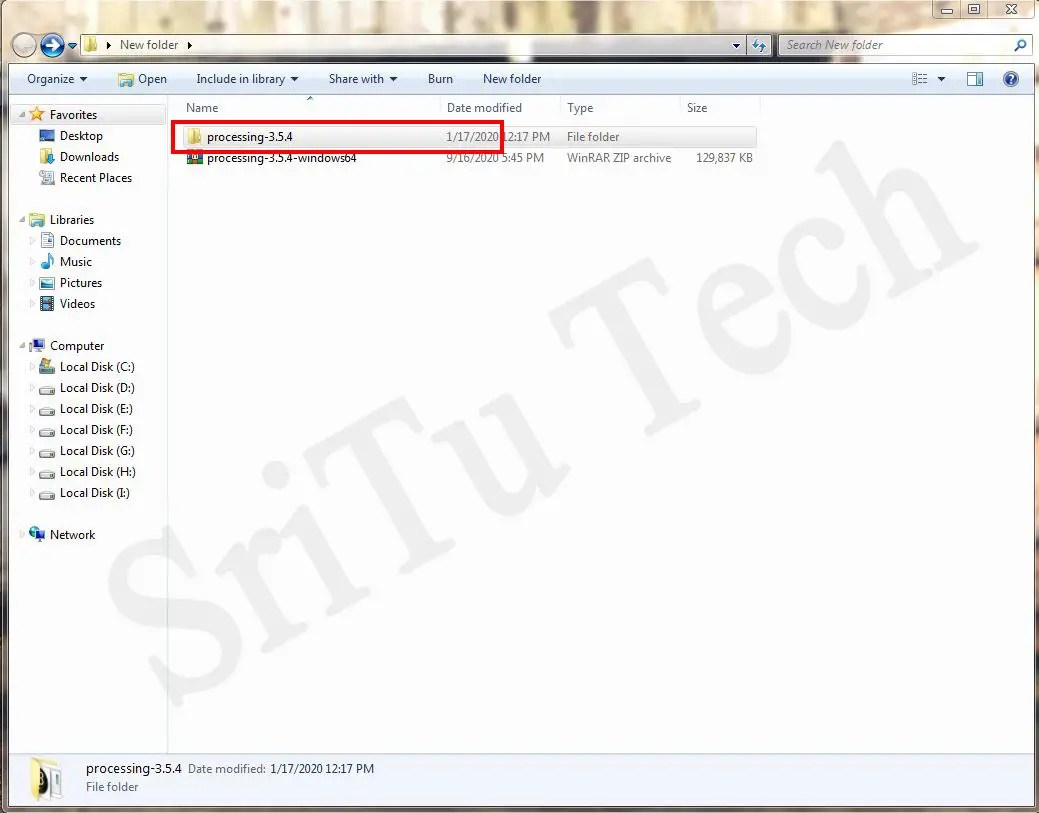Okay, now learned about the Processing IDE. So, now let’s do the project today. For that, the necessary components are given below.

### Components

OK, let’s do this project step by step.

### Step 1

First, let’s identify these components.

Arduino Nano board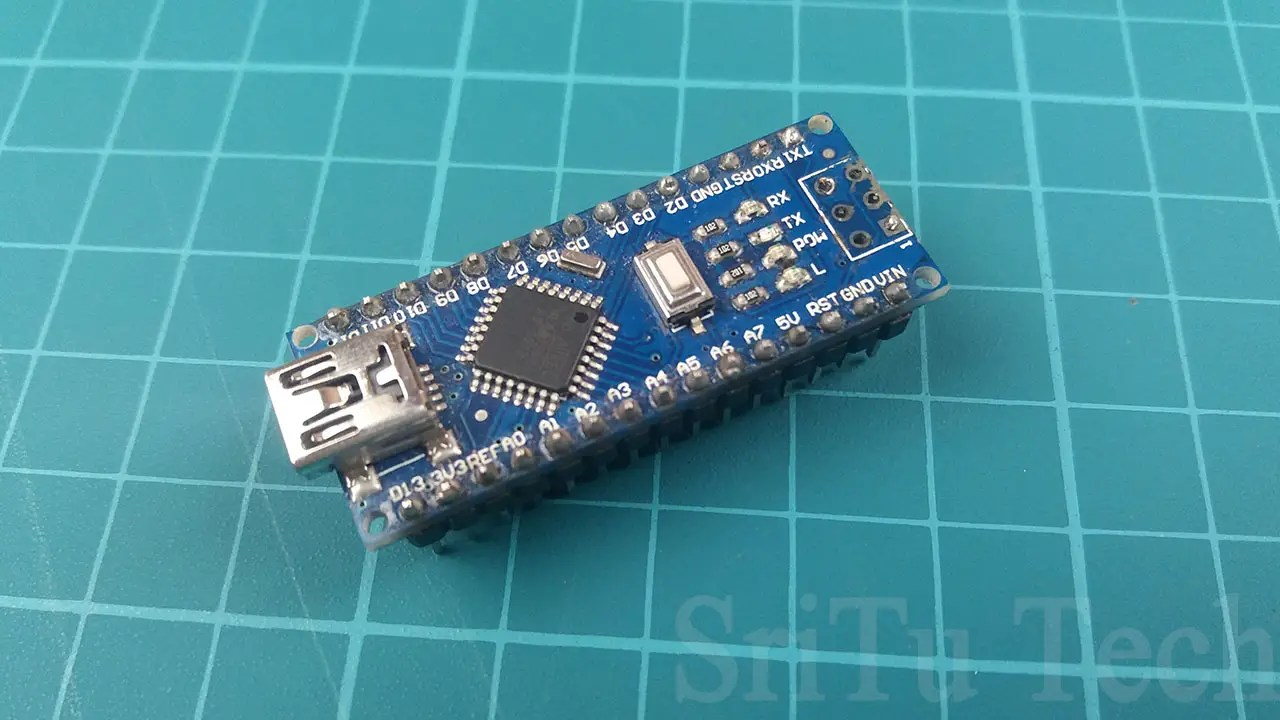You can use any other Arduino board.

LED bulbJumper wiresWe need two male-to-male jumper wires.Use this component to easily connect other components.

### Step 2

Next, connect these components using the circuit diagram below.Okay, now this work is done.

### Step 3

Now connect this Arduino Nano board to your computer using the USB cable. Let’s look at the code below.
``````/*LED on and off using the processing IDE.
created by the SriTu Tech team.
Read the code below and use it for any of your creation
*/
void setup() {
Serial.begin(9600);//enable serial communication
pinMode(2, OUTPUT);//define arduino pin
}
void loop() {
if (Serial.available() > 0) {//check serial value
if (led == '1') {//check serial read
digitalWrite(2, HIGH);//LED on
} else if (led == '0') {//check serial read
digitalWrite(2, LOW);//LED off
}
}
}``````
This code is very easy. The first time enabled serial communication to connect the processing IDE. Then, the second pin is set as the output pin. After using an if condition, the serial communication value is checked to see if it is 1 or 0. Finally, if the serial value is 1, an LED bulb is turned on and if the serial value is 0, the LED is turned off.

### Step 4

Then select the correct board and port and upload it.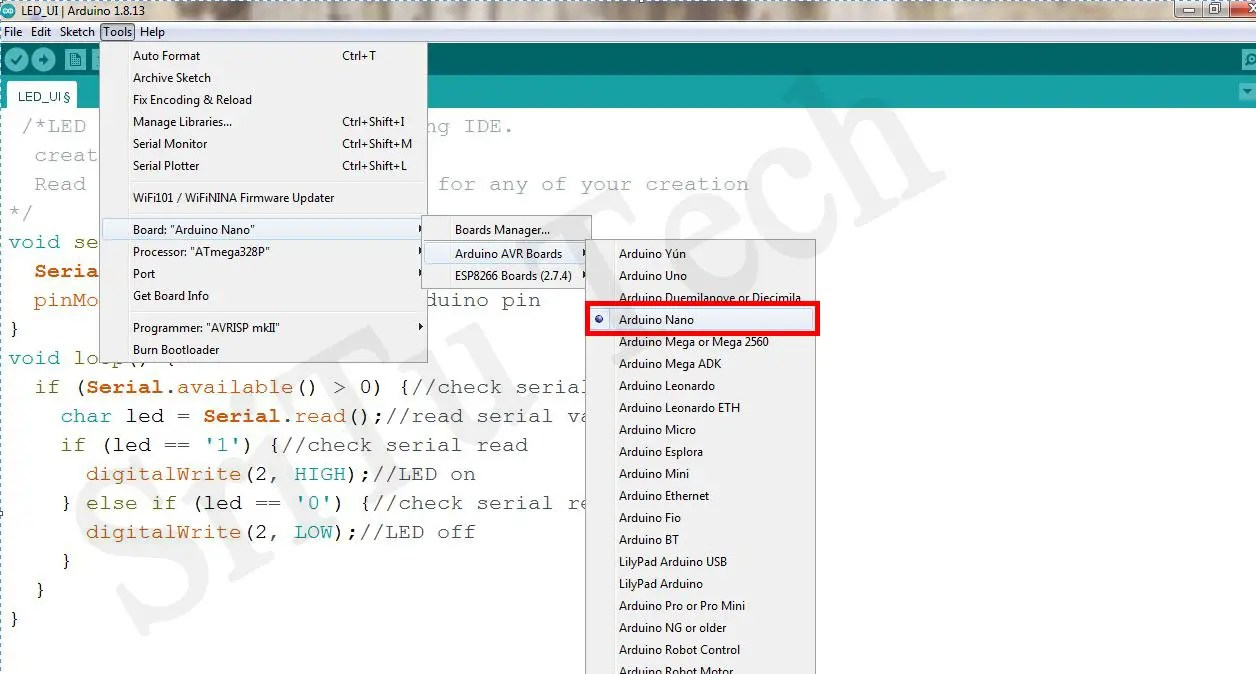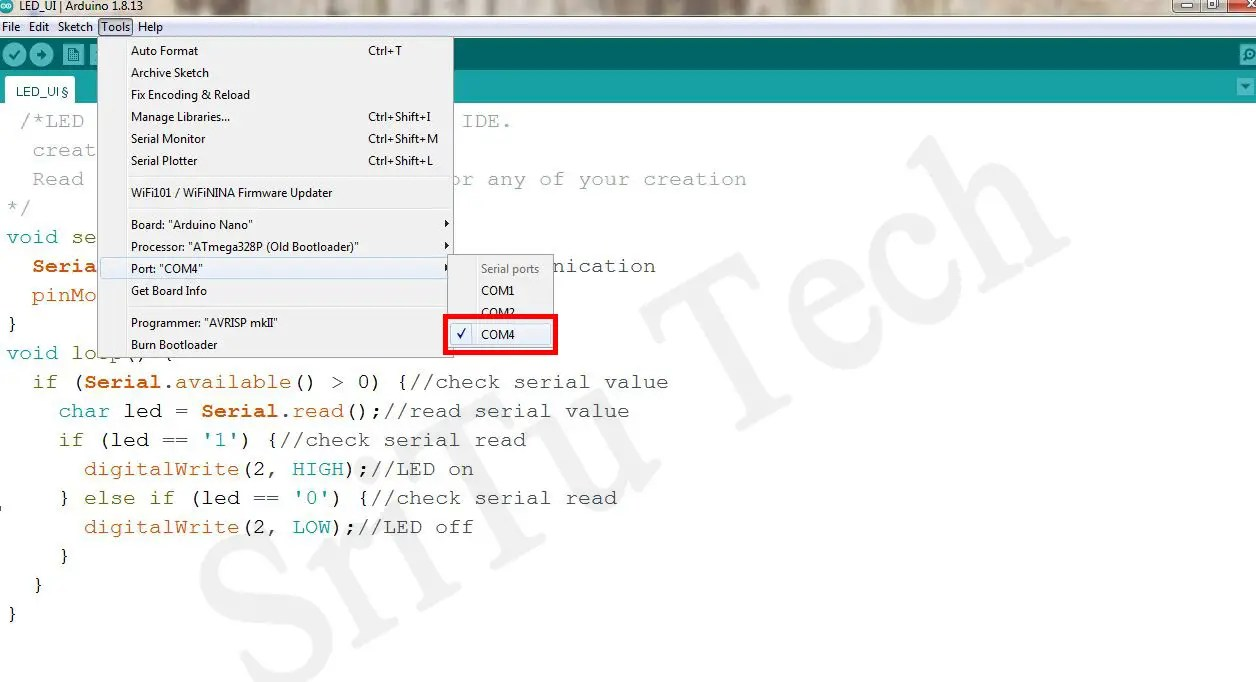### Step 5

OK, now let’s look at the processing code.
``````/*LED on and off using the processing IDE.
The second time uploads this code.
created by the SriTu Tech team.
Read the code below and use it for any of your creation
*/
import processing.serial.*;//import serial communicate library

Serial myPort;//create serial object

int circleOneX, circleOneY;      // Position of button one
int circleX, circleY;  // Position of button two
int circleOnesize = 90;     // Diameter button one
int circleSize = 90;   // Diameter of button two

color currentColor, baseColor;//color variable
color circleOnecolor, circleColor;//color variable
color circleOneHighlight, circleHighlight;//color variable

boolean circleOneOver = false;
boolean circleOver = false;

PFont f;//font type
void setup() {
size(300, 200);//screen size
circleOnecolor = color(0);//set colors to variable
circleOneHighlight = color(51);//set colors to variable
circleColor = color(255);//set colors to variable
circleHighlight = color(204);//set colors to variable
baseColor = color(102);//set colors to variable
currentColor = baseColor;

circleX = width/2+circleSize/2+25;
circleY = height/2;
circleOneX = width/2-circleOnesize+15;
circleOneY = height/2;

f = createFont("Ebrima Bold", 20);//Enter your font name
textFont(f);

String portName = "COM4";//Enter your COM port
myPort = new Serial(this, portName, 9600);//select first port
print(portName);
}

void draw() {
update(mouseX, mouseY);//main method
background(currentColor);//background color

if (circleOneOver) {
fill(circleOneHighlight);
} else {
fill(circleOnecolor);
}
stroke(255);
ellipse(circleOneX, circleOneY, circleOnesize, circleOnesize);//drow circle one

if (circleOver) {
fill(circleHighlight);
} else {
fill(circleColor);
}
stroke(0);
ellipse(circleX, circleY, circleSize, circleSize);//drow circle two

textAlign(LEFT);
fill(255);//black color
text("LED OFF", 37, 110);//display text

textAlign(RIGHT);
fill(0);//white color
text("LED ON", 255, 110);//display text

}

void update(int x, int y) {
if ( overCircle(circleX, circleY, circleSize) ) {
circleOver = true;
circleOneOver = false;
} else if (circleOneOver(circleOneX, circleOneY, circleOnesize) ) {
circleOneOver = true;
circleOver = false;
} else {
circleOver = circleOneOver = false;
}
}

void mousePressed() {
if (circleOver) {
currentColor = circleColor;
myPort.write('1');//send value one
print("LED on");
}
if (circleOneOver) {
currentColor = circleOnecolor;
myPort.write('0');//send value ziro
print("LED off");
}
}

boolean circleOneOver(int x, int y, int diameter) {
float disX = x - mouseX;
float disY = y - mouseY;
if (sqrt(sq(disX) + sq(disY)) < diameter/2 ) {
return true;
} else {
return false;
}
}

boolean overCircle(int x, int y, int diameter) {
float disX = x - mouseX;
float disY = y - mouseY;
if (sqrt(sq(disX) + sq(disY)) < diameter/2 ) {
return true;
} else {
return false;
}
}``````